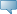## Detailed Course Information

Winter 2021
Oct 16, 2021Select the desired Level or Schedule Type to find available classes for the course.

 MATH 2160 - Numerical Analysis 1 (Lab required) Elementary techniques of numerical solution of mathematical problems: solution of equations, linear systems of equations, nonlinear equations; finite and divided differences, interpolation; numerical differentiation and integration. May not be held with MATH 2120, MATH 2161, the former MATH 2600, or the former MATH 2601. Prerequisites: [MATH 1232 (C) or MATH 1690 (C) or MATH 1700 (B) or MATH 1701 (B) or MATH 1710 (B)] and [MATH 1220 (C) or MATH 1300 (B) or MATH 1301 (B)]. - 3.0 Credit hours Science, Science lab Mathematics Department Course Attributes: Mathematics Requirement, Science Requirement for BA, Science Restrictions: Must be enrolled in one of the following Levels:            Undergraduate Must be enrolled in one of the following Campuses:            Main (Fort Garry & Bannatyne)